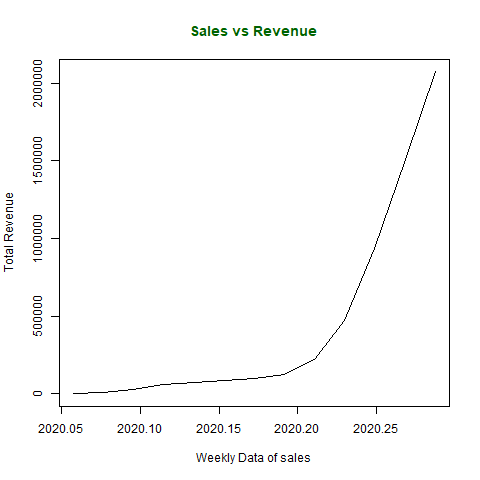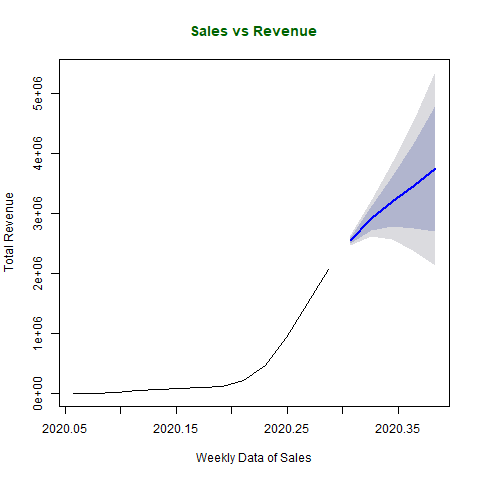# Predictive Analysis in R Programming

Predictive analysis in R Language is a branch of analysis which uses statistics operations to analyze historical facts to make predict future events. It is a common term used in data mining and machine learning. Methods like time series analysis, non-linear least square, etc. are used in predictive analysis. Using predictive analytics can help many businesses as it finds out the relationship between the data collected and based on the relationship, the pattern is predicted. Thus, allowing businesses to create predictive intelligence.

In this article, we’ll discuss the process, need and applications of predictive analysis with example codes.

#### Process of Predictive Analysis

Predictive analysis consists of 7 processes as follows:

• Define project: Defining the project, scope, objectives and result.
• Data collection: Data is collected through data mining providing a complete view of customer interactions.
• Data Analysis: It is the process of cleaning, inspecting, transforming and modelling the data.
• Statistics: This process enables validating the assumptions and testing the statistical models.
• Modelling: Predictive models are generated using statistics and the most optimized model is used for the deployment.
• Deployment: The predictive model is deployed to automate the production of everyday decision-making results.
• Model monitoring: Keep monitoring the model to review performance which ensures expected results.

#### Need of Predictive Analysis

• Understanding customer behavior: Predictive analysis uses data mining feature which extracts attributes and behavior of customers. It also finds out the interests of the customers so that business can learn to represent those products which can increase the probability or likelihood of buying.
• Gain competition in the market: With predictive analysis, businesses or companies can make their way to grow fast and stand out as a competition to other businesses by finding out their weakness and strengths.
• Learn new opportunities to increase revenue: Companies can create new offers or discounts based on the pattern of the customers providing an increase in revenue.
• Find areas of weakening: Using these methods, companies can gain back their lost customers by finding out the past actions taken by the company which customers didn’t like.

#### Applications of Predictive Analysis

• Health care: Predictive analysis can be used to determine the history of patient and thus, determining the risks.
• Financial modelling: Financial modelling is another aspect where predictive analysis plays a major role in finding out the trending stocks helping the business in decision making process.
• Customer Relationship Management: Predictive analysis helps firms in creating marketing campaigns and customer services based on the analysis produced by the predictive algorithms.
• Risk Analysis: While forecasting the campaigns, predictive analysis can show an estimation of profit and helps in evaluating the risks too.

Example:

Let us take an example of time analysis series which is a method of predictive analysis in R programming:

 `x <``-` `c(``580``, ``7813``, ``28266``, ``59287``, ``75700``,   ` `       ``87820``, ``95314``, ``126214``, ``218843``, ``471497``,  ` `       ``936851``, ``1508725``, ``2072113``)  ` `    `  `# library required for decimal_date() function  ` `library(lubridate)  ` `    `  `# output to be created as png file  ` `png(``file` `=``"predictiveAnalysis.png"``)  ` `    `  `# creating time series object  ` `# from date 22 January, 2020  ` `mts <``-` `ts(x, start ``=` `decimal_date(ymd(``"2020-01-22"``)),  ` `                             ``frequency ``=` `365.25` `/` `7``)  ` `    `  `# plotting the graph  ` `plot(mts, xlab ``=``"Weekly Data of sales"``,  ` `          ``ylab ``=``"Total Revenue"``,  ` `          ``main ``=``"Sales vs Revenue"``,   ` `          ``col.main ``=``"darkgreen"``)  ` `    `  `# saving the file   ` `dev.off()  `

Output:Forecasting Data:

Now, forecasting sales and revenue based on historical data.

 `x <``-` `c(``580``, ``7813``, ``28266``, ``59287``, ``75700``,   ` `       ``87820``, ``95314``, ``126214``, ``218843``,   ` `       ``471497``, ``936851``, ``1508725``, ``2072113``)  ` `    `  `# library required for decimal_date() function  ` `library(lubridate)  ` `    `  `# library required for forecasting  ` `library(forecast)  ` `    `  `# output to be created as png file  ` `png(``file` `=``"forecastSalesRevenue.png"``)  ` `    `  `# creating time series object  ` `# from date 22 January, 2020  ` `mts <``-` `ts(x, start ``=` `decimal_date(ymd(``"2020-01-22"``)),  ` `                            ``frequency ``=` `365.25` `/` `7``)  ` `    `  `# forecasting model using arima model  ` `fit <``-` `auto.arima(mts)  ` `    `  `# Next 5 forecasted values  ` `forecast(fit, ``5``)  ` `    `  `# plotting the graph with next   ` `# 5 weekly forecasted values  ` `plot(forecast(fit, ``5``), xlab ``=``"Weekly Data of Sales"``,  ` `ylab ``=``"Total Revenue"``,  ` `main ``=``"Sales vs Revenue"``, col.main ``=``"darkgreen"``)  ` `    `  `# saving the file   ` `dev.off()  `

Output:My Personal Notes arrow_drop_upCheck out this Author's contributed articles.

If you like GeeksforGeeks and would like to contribute, you can also write an article using contribute.geeksforgeeks.org or mail your article to contribute@geeksforgeeks.org. See your article appearing on the GeeksforGeeks main page and help other Geeks.

Please Improve this article if you find anything incorrect by clicking on the "Improve Article" button below.

Article Tags :

Be the First to upvote.

Please write to us at contribute@geeksforgeeks.org to report any issue with the above content.